Kinetic Theory of Gases: Assignment

# Kinetic Theory of Gases: Assignment Notes | Study Kinetic Theory & Thermodynamics - Physics

## Document Description: Kinetic Theory of Gases: Assignment for Physics 2022 is part of Kinetic Theory of Gases for Kinetic Theory & Thermodynamics preparation. The notes and questions for Kinetic Theory of Gases: Assignment have been prepared according to the Physics exam syllabus. Information about Kinetic Theory of Gases: Assignment covers topics like and Kinetic Theory of Gases: Assignment Example, for Physics 2022 Exam. Find important definitions, questions, notes, meanings, examples, exercises and tests below for Kinetic Theory of Gases: Assignment.

Introduction of Kinetic Theory of Gases: Assignment in English is available as part of our Kinetic Theory & Thermodynamics for Physics & Kinetic Theory of Gases: Assignment in Hindi for Kinetic Theory & Thermodynamics course. Download more important topics related with Kinetic Theory of Gases, notes, lectures and mock test series for Physics Exam by signing up for free. Physics: Kinetic Theory of Gases: Assignment Notes | Study Kinetic Theory & Thermodynamics - Physics
 1 Crore+ students have signed up on EduRev. Have you?

Q.1. One mole of gas is contained in cube of side 0.2m.if these molecules, each of mass 5 x 10-26kg, moves with translation speed 1 483ms-1 ,calculate the pressure exerted by the gas on the side of cube .

Time interval between successive collision .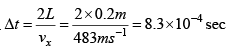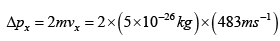=  4.83 x1023 N-S

Force exerted for one molecule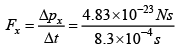= 0.582 x 10-19N

Total force exerted by one mole gas is ( 6 x1023 ) x ( 0.582 x 10-19 N ) = 3.49 x 104N

Average pressure is given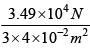= 2.95 x 105 NM-2

Q.2. Calculate the fraction of gas molecules which have the mean–free path in the range λ to 2λ .

The fraction of gas molecules that do not undergoes collision after path length x is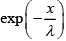. Therefore the fraction of molecules that has free path values between  λ to 2λ is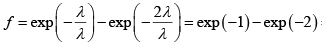= 0.37 - 0.14 = 0.23

Q.3. The molecules of a gas move an average speed of 1 450ms-1 . If  η = 16.6 x 10-6 Nsm-2, ρ = 1.25kgm-3 and n = 2.7 x 1025 m-3. Calculate the mean free path and diameter of the gas molecules.

We can write as

λ =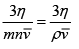On inserting the given numerical values, we get

λ =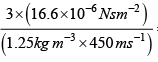= 8.85 x 10-8m

λ =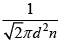We can invert this relation to express d in terms of λ :

d =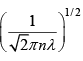=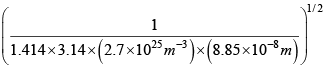= 3.42 x 10-10m

Q.4. (a) Three closed vessel A, B and C at the same temperature T and contains gases which obey the MB distribution of velocities. Vessel A contains only O2 , B only N2 and C a mixture of equal quantities O2 and N2 . If the average speed of the O2 molecules in vessel A is v1 , that of N2 molecules in vessel B in v2 , calculate the average speed of the molecules in vessel C

(b) The temperature of an ideal gas is increased from 120K to 480K . The rms velocity of the gas molecules is v , at 480K Calculate the rms velocity of the gas molecule.

(a) The average speed of molecules of an ideal gas is given by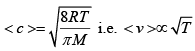for same gas.

Since, the percentage of A & C are same, average speed of O2 molecules will be equal in A & C i.e. v1 .

(b)=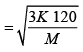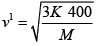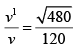v1 = 2v

Q.5. (a) Two identical containers A and B with friction less pistons contain the same ideal gas at the same temperature and same volume V . The mass of the gas in A is mA and that in B is mB . The gas in each cylinder is allowed to expand isothermally to the same volume 2V . The changes in the pressure in A & B are found to be ΔP and 1.5ΔP respectively. Then find the ratio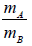(b) Find the ratio of the speeds of sound in nitrogen gas to that in helium gas, at 300K

(a) Process is isothermal. Therefore, T = constt ×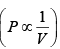volume is increasing, therefore pressure will decrease.

In Chamber (A)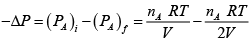=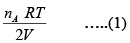In Chamber (B)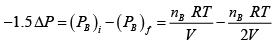=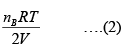From (1) & (2)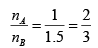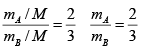3mA = 2mB

(b) v =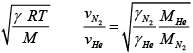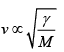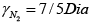=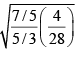= √3/5

Q.6. Plot the Density of states as a function of energy and momentum in three, two and one dimensional systems.

(a) 3D

(b) 2D

(c) 1D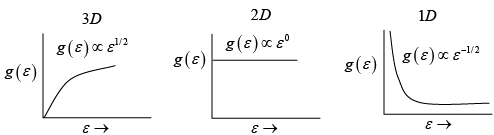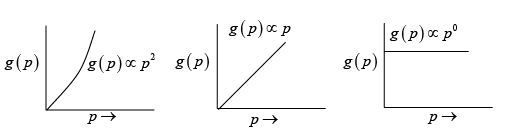Q.7. In the interstellar space, the density of hydrogen molecule H2 is about one molecule / cm3 .Taking the diameter of H2 approximately 1A0 , calculate

(a) Average velocity of the molecule at kinetic temperature is 100K

(b) Mean free path of the Hmolecules

(c) Collision frequency

ρ = 1 molecule / cm3 diameter d = 1A0 T = 300K

m  = 2x (1.67 x 10-24) = 3.34 x 10-24 gm

(a) Average velocity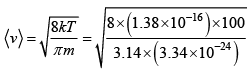= 1 x 105cm/sec

(b)  Mean free path λ =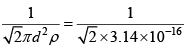= 2 x 1015 cm

(c) v =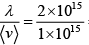= 2 collision per second

Q.8. The mean free path of the molecules of a gas at a pressure p and temperature T is 3x107m . Calculate the mean free path if

(a) the temperature is doubled and

(b) the pressure is doubled.

(a) We know

λ =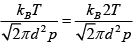When the temperature is doubled, we note that the mean free path will increase by a factor of two. Hence,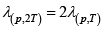= 6 x10-7 m

(b) When the pressure is doubled, the mean free path will be halved: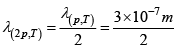= 1.5 x10-7 m

Q.9. (a) At the room temperature, the rms speed of the molecules of a certain diatomic gas is found to be 1930m/ s . What is the name of the gas?

(b) If one mole of a monatomic gas (γ = 5/ 3) is mixed with one mole of a diatomic gas (γ = 7/ 5) . Calculate the value of γ for the mixture?

(a)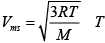= 300K

1930m/s =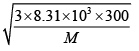M = 2 g/ mol or the gas is H.

(b) γ1 = 5/3 means gas monatomic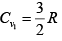γ2 = 7/5 means gas diatomic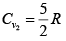Cv (for mixture) =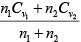=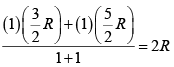Cp = Cv + R = 3R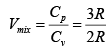= 1.5

Q.10. (a) The average translational energy and the rms speed of molecules in a sample of oxygen gas at 300K are 6.21x10-21 J & 484m/ s respectively. The corresponding values at 600K are nearly (assuming ideal gas behavior)

(b) The average translational energy of O2 (molar mass 32) molecules at a particular

temperature is 0.048eV . Calculate the translation K.E. of N2 (molar mass 28 ) molecules in eV at the same temperature?

(c) A vessel contains 1mole of O2 gas (molar mass 32) at a temperature T . The pressure of the gas is P' . An identical vessel containing one mole of the gas (molar mass 4 ) at a temperature 2T , Calculate the pressure.

(d) A vessel contains a mixture of one mole of O2 & two moles of N2  at 300K .

Calculate the ratio of the average rotational kinetic energy per O2  molecule to per Nmolecule.

(a)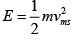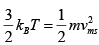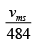= √2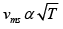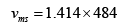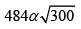= 684.37m/s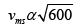K.E = 6.21 x 2 x10-21 J

= 12.42 x 10-21 J

(b) Average translational energy of an ideal gas molecule is 3/2kT which depends on temperature only, Therefore if same temperature 0.048eV

(c) PV = μ RT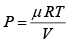P ∝ T

P' = 2P

(d) Average kinetic energy per molecule per degree of freedom= 1/2kT. Since, both the

gases are diatomic and at same temperature ( 300K ), both will have same rotational DOF = 2 therefore, both the gases will have the same average rotational K ×E× per molecule

=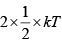= kT

The document Kinetic Theory of Gases: Assignment Notes | Study Kinetic Theory & Thermodynamics - Physics is a part of the Physics Course Kinetic Theory & Thermodynamics.
All you need of Physics at this link: Physics

## Kinetic Theory & Thermodynamics

20 docs|32 tests
 Use Code STAYHOME200 and get INR 200 additional OFF

## Kinetic Theory & Thermodynamics

20 docs|32 tests

Track your progress, build streaks, highlight & save important lessons and more!

,

,

,

,

,

,

,

,

,

,

,

,

,

,

,

,

,

,

,

,

,

;